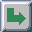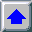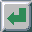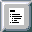Next: Conclusion and Future Work Up: Incident Light Metering Previous: Radiosity

# Color Case

The incident light metering has so far been described for the monochrome case. In reality the diffusors gather colored light from half spaces. Color light can either be caused by color light sources or can be the result of interreflections at "color" BRDFs.

The color case should include the monochrome case as a special case (here monochrome means that r=g=b, or in the general case the spectral reflectivity, as neutral color and lighting. The results should then be the same as in the monochrome case.

It is possible to introduce more color models for irradiance computation which satisfy these conditions. These methods are very similar under average lighting conditions, but they can differ a lot when used in highly saturated color lighting (e.g. disco, color neon, theater, fireworks lighting etc.).

Our intention for the color case is to find a scalar value f that represents the irradiance value of the full spectrum. When using a simple 3 channel model (for lighting and for BRDFs) for a given rgb triplet this value f can be: f(r,g,b)=Y(r)+Y(g)+Y(b), where Y represents the CIE Y value which corresponds to the relative lightness and has a maximum value of 100. But also other functions like f(r,g,b)=(r+g+b)/3 or f(r,g,b)=max(r,g,b) are appropriate for the neutral color. In the last two models the neutral color is represented by an rgb triplet where r=g=b. Which formula from these three should be used? The Y approach is widely used in photography, in spite of its drawbacks. As stated before this approach gives good results for average lighting color conditions. Figure 7.4 shows the ideal (human eye) and the real spectral sensitivity curve of silicon blue cells used in an incident light meter.Figure 7.4: Spectral sensitivity of human eye and Si blue cell

To compare the three suggested scalar functions f(r,g,b) we take a sample scene that consists of a homogeneously lit square, which lies normal to the viewing direction. One quarter of this square is medium red, i.e., and (r,g,b)=(0.5,0,0), the second quarter is medium green, i.e., (r,g,b)=(0,0.5,0), the third quarter is medium blue, i.e., (r,g,b)=(0,0,0.5), and finally the last quarter is neutral, medium gray, i.e., (r,g,b)=(0.5,0.5,05).

For neutral homogeneous lighting (r,g,b)=(1,1,1) irradiance metering results in a neutral color which should only be scaled with Y=100 or (r,g,b)=(1,1,1) (for the sake of simplicity, the irradiance to radiance conversion will not be explained, we only want to illustrate the original color shift). The resulting colors will be the same as the original, medium red, medium green, medium blue and medium gray.

Let us see what happens for a blue lighting (0,0,1). With light metering we will measure an irradiance of Y=10, so we should use a scaling factor of 100/10=10, which will make all colors highly (10 times) over-exposed. More precisely, the red and green square quarters will be black as they have no blue component, but the blue and gray square quarters will result in (r,g,b)=(0,0,5) which will be displayed as (0,0,1) after the clipping instead of the correct (0,0,0.5).

For green lighting, over-exposure will still occur, with (r,g,b)=(0,0.71,0) for the green and gray square quarter, since green has the biggest Y value, and blue the smallest (see fig. 7.4).

When f(r,g,b)=(r+g+b)/3 is used as an irradiance measuring formula, for neutral (white) light the result is the same as above. For green and blue lighting the resulting values are the same (only the corresponding components are not). For this formula, green will be lighter than before, but blue will not be so highly over-exposed. The scale factor will be 3 for both lightings, so instead of the correct 0.5 the corresponding components will be 1.5, i.e. 1 after clipping. The zeros will remain zeros. It is obvious that the above two methods sometimes produce significant errors.

Finally, the third function f(r,g,b)=max(r,g,b) will be examined. The idea is to compute the (r,g,b) irradiance value for each diffusor and to store the maximum value for later median determination. In the above example, with white, green and blue lighting the new (fictive) irradiance value will be 1, and the scale factor will be 1 as well. For white lighting the results are correct just as before. With blue lighting the red and green square quarters will be black (which is the correct result), and the medium blue and the medium gray square quarters will be correctly displayed as (0,0,0.5) medium blue.

The results will also be correct for all square quarters with green and red lightings. We suggest the reader finds scale factors for various combinations of lighting (yellow, purple, etc.) and for various color samples. This method always gives correct or significantly better results than the first two approaches.

In the general case when there are K (K>3) discrete wavelengths, the appropriate (r,g,b) triplet should be computed first. This can be done by computing CIE XYZ coordinates first, and then converting them to r, g, b.

Sometimes one of the r, g, or b values can become negative, which means that the color is outside of the device's gamut. At least one of the r, g, b values will always be positive, however, so the selected maximum f(r, g, b)=max(r,g,b) (fictive) irradiance is always positive.

For neutral lighting the new maximum method gives the same result, for unsaturated lighting it gives a similar result and for saturated lighting it gives significantly better results than the "Y approach" widely used in photography.

The incident light metering method is ilustrated in the results chapter, color plates 9, 10, 11, 12, 13, 14, 15, 16, and 17.Next: Conclusion and Future Work Up: Incident Light Metering Previous: Radiosity

matkovic@cg.tuwien.ac.at